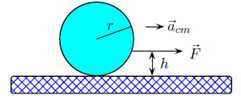Problem: A ball of radius r with a wire glued to one spot on its surface can be pulled along the floor and will slide without any tendency to roll only if the wire is horizontal and is a distance h above the floor. In terms of F, the magnitude of the applied force, fk, the magnitude of the force of kinetic friction, and the radius r of the ball, what is h? 1. h = r (f k − F) 2. h = 0; the wire must run along the floor. 3. h = r (f k/F) 4. h = r 5. h = r (1 + F/f k) 6. h = r (F − f k) 7. h = r (F/f k) 8. h = r (1 − f k/F) 9. h = r (1 + f k/F) 10. h = r (1 −F/f k)

85% (21 ratings)
Problem Details

A ball of radius r with a wire glued to one spot on its surface can be pulled along the floor and will slide without any tendency to roll only if the wire is horizontal and is a distance h above the floor. In terms of F, the magnitude of the applied force, fk, the magnitude of the force of kinetic friction, and the radius r of the ball, what is h?

1. h = r (f k − F)

2. h = 0; the wire must run along the floor.

3. h = r (f k/F)

4. h = r

5. h = r (1 + F/f k)

6. h = r (F − f k)

7. h = r (F/f k)

8. h = r (1 − f k/F)

9. h = r (1 + f k/F)

10. h = r (1 −F/f k)